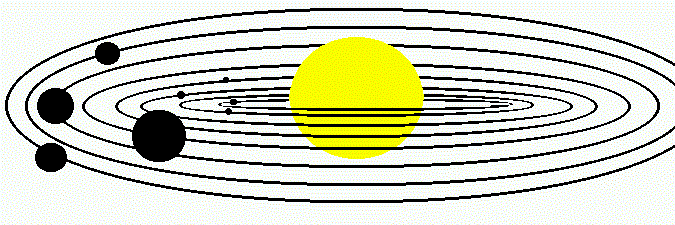## Teacher Area-Spin Through Solar System## Materials:

• At least one computer connected to the Internet with software to view GIF images
• A laser or inkjet printer
• Blank transparency acetates or tracing paper for each group of students
• A compass for each group of students
• A protractor for each group of students
• A calculator (with trig functions for advanced students)
• A stopwatch for each group of students
• A tangerine or an orange for each group of students
• A basketball tied to the ceiling (easier said than done) or a globe

## Objectives:

• To pull together current information and the latest images of Solar System from the Internet

• To refresh such concepts as ratios, circles, angles, arcs, angular velocity, and period of rotation

• To demonstrate the application of math concepts to astronomy

• To introduce and discuss general information about the structure of and planetary motion in the Solar System

## Directions:

### There are two ways of working with this unit. The first one is more traditional. You will:

1. Introduce new material in class

2. Explain how the rotation rates of planets can be calculated

3. Refresh math concepts and formulas, such as: What is ratio? How to measure an arc in a circle? What is angular velocity?

4. Use one planet as an example

5. Show step by step how such work (including research, calculations, and conclusions) should be organized

6. Discuss the results and give instructions for other planets

7. Print out some images and data, and pass them out for a homework assignment

8. Organize summary discussion and give hints to students on how to make conclusions

If time for this unit is limited, you can organize group work by assigning images and data concerning different planets to different groups. At the end of the unit small groups will present their calculations in front of the class, and then discuss similarities and differences between various planets.

Another approach to this interesting material is merely to supervize students' research by giving them short instructions on how to work with the Self-Study Guide, and by answering questions if there are any.

At the end of the unit students will be able to answer the questions from the work sheet. You can use it as a pre-test, post-test, or both.

To teach the angular velocity section, you may want to use a globe or a basketball tied with twine and hung up so that you can spin it. It can be used to demonstrate to students how to figure out the time it takes to make one turn.

You can also try turning the lights on and off to simulate seeing only part of the planet's rotation. You could even put some "clouds" between your young astronomers and the planet "Spaulding."